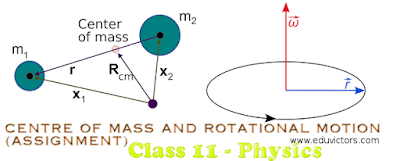## CENTRE OF MASS AND ROTATIONAL MOTION (ASSIGNMENT)

1. Two bodies of masses 0.5 kg and 1 kg are lying in XY plane at (-1,2) and (3,4) respectively. What are the co-ordinates of the centre of mass?

2. Three points masses of 1 kg, 2 kg and 3 kg lie a (1,2), (0,-1) and (2,-3) respectively. Calculate the co-ordinates of the centre of mass of the system.

3. Three identical spheres each of radius r, and mass m are placed touching each other on a horizontal floor. Locate the position of centre of mass of the system.

4. Two particles of mass 2 kg and 1 kg are moving along the same line with speeds 2m/s and 5m/s respectively. What is the speed of the centre of mass of the system. if both the particles are moving
(a) in same direction
(b) in opposite direction?

Answer: 3m/s, 1/3 m/s in the direction of 1 kg

5. Consider a two-particle system with the particles having masses, m 1 and m 2 . If the first particle is pushed towards the centre of mass through a distance d, by what distance should the second particle be moved so as to keep the centre of mass at the same position.

6. The centre of gravity of a loaded taxi is 1.5 m above the ground, and the distance between the wheels is 2 m. What is the maximum speed with which it can go round and unbanked curve of radius 100 m without being turned upside down. What minimum value of coefficient of friction is needed at this speed?

7. A threaded rod with 12 turns/cm and diameter 1.18 cm is mounted horizontally. A bar with a threaded hole to match the rod is screwed onto the rod. The bar spins at 216 rev/min.How long will it take for the bar to move 1.50 cm along the rod?

8. A particle starts rotating from rest according to the formula θ = 3t³/20 – t²/3. Calculate the angular velocity and angular acceleration at the end of 5 second.

9. Three points masses m 1 , m 2 and m 3 are located at the vertices of an equilateral triangle of length a. Calculate the moment of inertia of the system about an axis along the altitude of the triangle passing through m 1.

10. The sun rotates around itself once in 27 days. If it were to expand to twice its present diameter, what would be its new period of revolution?

11. A child stands at the centre of a turn table with its two arms outstretched. The turn table is set rotating with frequency of 40 r.p.m. How much is the frequency of revolution of the child if he folds his hands back and hereby reduces his moment of inertia to 2/5 times the initial value? Assume that the turn table rotates without friction.

12. A spherical ball rolls on a table without slipping. Determine the percentage of its K.E. which is rotational. Moment of inertia of sphere =2/5 mr²

13. A solid cylinder rolls down an inclined plane. Its mass is 2 kg and radius 0.1 m. If the height of the inclined plane is 4 m, what is its rotational kinetic energy when it reaches the foot of the plane? Assume that the surface are smooth.

14. Two small balls, A and B, each of mass m, are attached rigidly to the ends of a light rod of length d. The structure rotates about the perpendicular bisector of the rod at an angular speed ω. Calculate the angular momentum of the individual balls and of the system about the axis of rotation.

15. A cylinder of mass 5kg is rolling on a plane of inclination 30 0 . Find the force of friction between the cylinder and the surface of inclined plane.

16. Prove that for an earth satellite, the ratio of velocity at apogee (when farthest from earth) to its velocity at perigee (when nearest to earth) is equal to the inverse ratio of its distance from apogee and perigee.